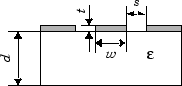# txlineCPW

Create coplanar waveguide transmission line

## Description

Use the `txlineCPW` object to create a coplanar waveguide transmission line. A cross-section of a coplanar waveguide transmission line is shown in this figure. The physical characteristics of the coplanar waveguide transmission line include the conductor width (w), the conductor thickness (t), the slot width (s), the substrate height (d), and the permittivity constant (ε).## Creation

### Syntax

``txline = txlineCPW``
``txline = txlineCPW(Name,Value)``

### Description

````txline = txlineCPW` creates a default coplanar waveguide transmission line object.```

example

````txline = txlineCPW(Name,Value)` sets Properties using one or more name-value pairs. For example, `txline = txlineCPW('SlotWidth',0.0046)` creates a coplanar waveguide transmission line with a slot width of `0.0046` meters.```

## Properties

expand all

Name of the coplanar waveguide transmission line, specified as a string scalar or a character vector.

Example: `'Name','cpw1'`

Example: `txline.Name = 'cpw1'`

Data Types: `char` | `string`

Physical width of the conductor, specified as a positive scalar in meters.

Example: `'ConductorWidth',0.0200`

Example: `txline.ConductorWidth = 0.0200`

Data Types: `double`

Physical width of the slot, specified as a positive scalar in meters.

Example: `'SlotWidth',0.0008`

Example: `txline.SlotWidth = 0.0008`

Data Types: `double`

Physical height of the conductor or dielectric thickness, specified as a positive scalar in meters.

Example: `'Height',0.000835`

Example: `txline.Height = 0.000835`

Data Types: `double`

Physical thickness of the transmission line, specified as a positive scalar in meters.

Example: `'Thickness',0.000008`

Example: `txline Thickness = 0.000008`

Data Types: `double`

Relative permittivity of the dielectric, specified as a positive scalar.

Example: `'EpsilonR',8.8`

Example: `txline.EpsilonR = 8.8`

Data Types: `double`

Loss angle tangent of the dielectric, specified as a nonnegative scalar.

Example: `'LossTangent',1`

Example: `txline.LossTangent = 1`

Data Types: `double`

Conductivity of the conductor, specified as a nonnegative scalar in Siemens per meter (S/m).

Example: `'SigmaCond',2`

Example: `txline.SigmaCond = 2`

Data Types: `double`

Physical length of the transmission line, specified as a positive scalar in meters.

Example: `'LineLength',0.0200`

Example: `txline.LineLength = 0.0200`

Data Types: `double`

Stub transmission line termination, specified as `'NotApplicable'`, `'Open'`, or `'Short'`.

Example: `'Termination','Short'`

Example: `txline.Termination = 'Short'`

Data Types: `char`

Type of stub, specified as `'NotAStub'`, `'Series'`, or `'Shunt'`.

Example: `'StubMode','Series'`

Example: `txline.StubMode = 'Series'`

Data Types: `char`

Number of input and output ports, returned as positive scalar.

Data Types: `double`

Terminals of the coplanar waveguide transmission line, returned as a cell array of strings.

Data Types: `char` | `string`

## Object Functions

 `sparameters` S-parameter object `groupdelay` Group delay of S-parameter object or RF filter object or RF Toolbox circuit object `noisefigure` Calculate noise figure of transmission lines `getZ0` Calculate characteristic impedance of transmission line object `circuit` Circuit object `clone` Create copy of existing circuit element or circuit object

## Examples

collapse all

Create a coplanar waveguide transmission line using these specifications:

• Conductor width : 45 mu m

• Slot width : 50 mu m

• Height of substrate : 525 mu m

• Thickness : 1 mu m

• GaAS permittivity or Epsilon R : 2.5 F/m

• Conductivity : 3.33e7 S/m

```cpwtxline = txlineCPW('ConductorWidth',45e-6,'SlotWidth',50e-6,'Height',525e-6,... 'Thickness',1e-6,'EpsilonR',2.5,'SigmaCond',3.33e7)```
```cpwtxline = txlineCPW: CPW element Name: 'CPW' ConductorWidth: 4.5000e-05 SlotWidth: 5.0000e-05 Height: 5.2500e-04 Thickness: 1.0000e-06 EpsilonR: 2.5000 LossTangent: 0 SigmaCond: 33300000 LineLength: 0.0100 Termination: 'NotApplicable' StubMode: 'NotAStub' NumPorts: 2 Terminals: {'p1+' 'p2+' 'p1-' 'p2-'} ```

Calculate the S-parameters of the transmission line at 20 GHz.

`sparam = sparameters(cpwtxline,20e9);`